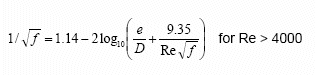# Friction Factor Calculations

The Darcy-Weisbach equation, for calculating the friction loss in a pipe, uses a dimensionless value known as the friction factor (also known as the Darcy-Weisbach friction factor or the Moody friction factor) and it is four times larger than the Fanning friction factor.

## Friction Factor Chart / Moody Chart

The friction factor or Moody chart is the plot of the relative roughness (e/D) of a pipe against the Reynold's number. The blue lines plot the friction factor for flow in the wholly turbulent region of the chart, while the straight black line plots the friction factor for flow in the wholly laminar region of the chart.In 1944, LF Moody plotted the data from the Colebrook equation and the resulting chart became known as The Moody Chart or sometimes the Friction Factor Chart. It was this chart which first enabled the user to obtain a reasonably accurate friction factor for turbulent flow conditions, based on the Reynolds number and the Relative Roughness of the pipe.

## Friction Factor for Laminar Flow

The friction factor for laminar flow is calculated by dividing 64 by the Reynold's number.

Friction factor (for laminar flow) = 64 / Re

## Critical Flow Condition

When flow occurs between the Laminar and Turbulent flow conditions (Re 2300 to Re 4000) the flow condition is known as critical and is difficult to predict. Here the flow is neither wholly laminar nor wholly turbulent. It is a combination of the two flow conditions.

## Friction Factor for Turbulent Flow

The friction factor for turbulent flow is calculated using the Colebrook-White equation:

### Colebrook-White EquationDue to the implicit formation of the Colebrook-White equation, calculation of the friction factor requires an iterative solution via numerical methods.

The friction factor is then used in the Darcy-Weisbach formula to calculate the fluid frictional loss in a pipe.

Secure Online PaymentsPipe Flow Software: Piping design, Pressure drop calculator, Flow rate calculator, Pump head calculations, Pump selection software.  Copyright © Pipe Flow Software 1997-2023